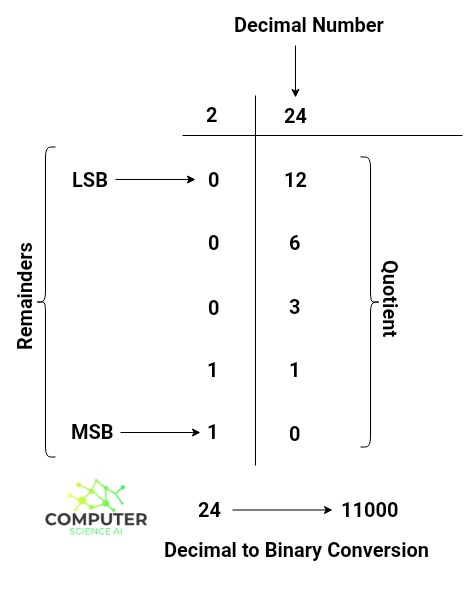# Decimal to Binary Converter

Enter a decimal number

Binary number (Base 2):

Binary from signed 2's complement (Base 2):

Hex number (Base 16):

## Decimal To Binary Conversion

A decimal number system is a base 10 number system. It means that a decimal number can be formed by using any of the 0-9 digits (10 base elements). On the other hand, the binary number system has base 2. It means that a binary number can be formed by using 0 and 1 (2 base elements) only.

To convert a decimal number into binary value you need to divide the decimal number continuously by 2.

The diagram below explains decimal to binary conversion process :In above diagram LSB stands for Least Significant Bit and MSB stands for Most Significant Bit. To get binary value corresponding to given decimal we need to divide it continuously by 2 until we get 0 as quotient.

After the division process stops, look for the remainders write them starting from the MSB.

So 24 in decimal is equivalent to 11000 in binary number system.